# 你确定(a == 1 && a == 2 && a == 3)不能为true?FE @ 公众号: 前端胖头鱼

## 前言## 障眼法我TM给跪了

### 解法1：隐藏字符 + if

``````
const if‌ = () => !0
const a = 9

if‌(a == 1 && a == 2 && a == 3)
{
console.log('前端胖头鱼') // 前端胖头鱼
}

``````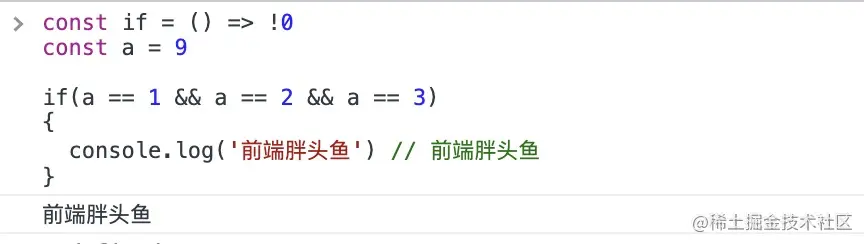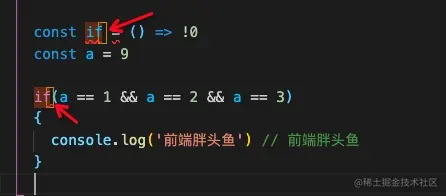`真相大白`if的后面有个隐藏字符，本质上是声明了一个无论输入啥都返回true函数，而下面的代码块，更是和这个函数没半毛钱关系，怎么样都会执行!!!

``````
{
console.log('前端胖头鱼') // 前端胖头鱼
}
``````

### 解法2：隐藏字符 + a变量

``````const aﾠ = 1
const a = 2
const ﾠa = 3

if (aﾠ == 1 && a == 2 && ﾠa == 3) {
console.log('前端胖头鱼') // 前端胖头鱼
}

``````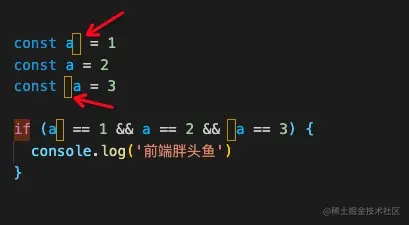### 解法3：隐藏字符 + 数字变量

``````const a = 1
const ﾠ1 = a
const ﾠ2 = a
const ﾠ3 = a

if (a == ﾠ1 && a == ﾠ2 && a == ﾠ3) {
console.log('前端胖头鱼') // 前端胖头鱼
}
``````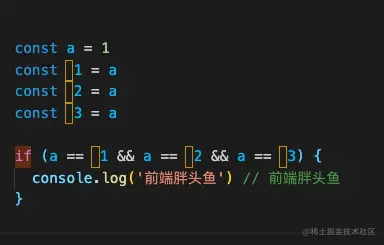## 再来一种奇特的解法

### 解法4：“with”

MDN上映入眼帘的是一个警告，仿佛他的存在就是个错误，我也从来没有在实际工作中用过他，但他却可以用来解决这个题目。``````let i = 1

with ({
get a() {
return i++
}
}) {
if (a == 1 && a == 2 && a == 3) {
console.log('前端胖头鱼')
}
}

``````

## 隐式转换成解题的关键

### 隐式转换部分规则

JS中使用`==`对两个值进行比较时，会进行如下操作：

1. 将两个被比较的值转换为相同的类型。
2. 转换后（等式的一边或两边都可能被转换）再进行值的比较。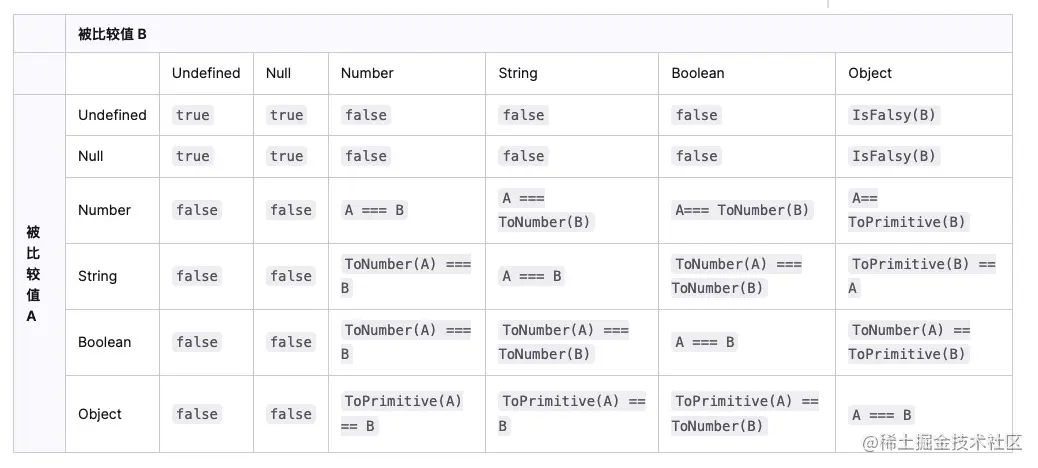1. a类型为String，并且可转换为数字1（`'1' == 1 => true`
2. a类型为Boolean，并且可转换为数字1 (`true == 1 => true`)
3. a类型为Object，通过`转换机制`后，可转换为数字1 （`请看下文`

### 对象转原始类型的"转换机制"

1. 如果有Symbol.toPrimitive方法，优先调用再返回，否则进行2。
2. 调用valueOf，如果可以转换为原始类型，则返回，否则进行3。
3. 调用toString，如果可以转换为原始类型，则返回，否则进行4。
4. 如果都没有返回原始类型，会报错。
``````const obj = {
value: 1,
valueOf() {
return 2
},
toString() {
return '3'
},
[Symbol.toPrimitive]() {
return 4
}
}

obj == 4 // true
// 您可以将Symbol.toPrimitive、toString、valueOf分别注释掉验证转换规则

``````

### 解法5: Symbol.toPrimitive

``````const a = {
i: 1,
[Symbol.toPrimitive]() {
return this.i++
}
}
// 每次进行a == xxx时都会先经过Symbol.toPrimitive函数，自然也就可以实现a依次递增的效果
if (a == 1 && a == 2 && a == 3) {
console.log('前端胖头鱼') // 前端胖头鱼
}

``````

### 解法6: valueOf vs toString

``````let a = {
i: 1,
// valueOf替换成toString效果是一样的
// toString
valueOf() {
return this.i++
}
}

if (a == 1 && a == 2 && a == 3) {
console.log('前端胖头鱼') // 前端胖头鱼
}

``````

### 解法7：Array && join

``````let a = [1, 2, 3]

a.join = a.shift

if (a == 1 && a == 2 && a == 3) {
console.log('前端胖头鱼') // 前端胖头鱼
}

``````

## 数据劫持亦是一条出路

### 解法8：Object.defineProperty

``````let _a = 1
Object.defineProperty(window, 'a', {
get() {
return _a++
}
})

if (a == 1 && a == 2 && a == 3) {
console.log('前端胖头鱼') // 前端胖头鱼
}

``````

### 解法9：Proxy

``````let a = new Proxy({ i: 1 }, {
get(target) {
return () => target.i++
}
})

if (a == 1 && a == 2 && a == 3) {
console.log('前端胖头鱼') // 前端胖头鱼
}

``````